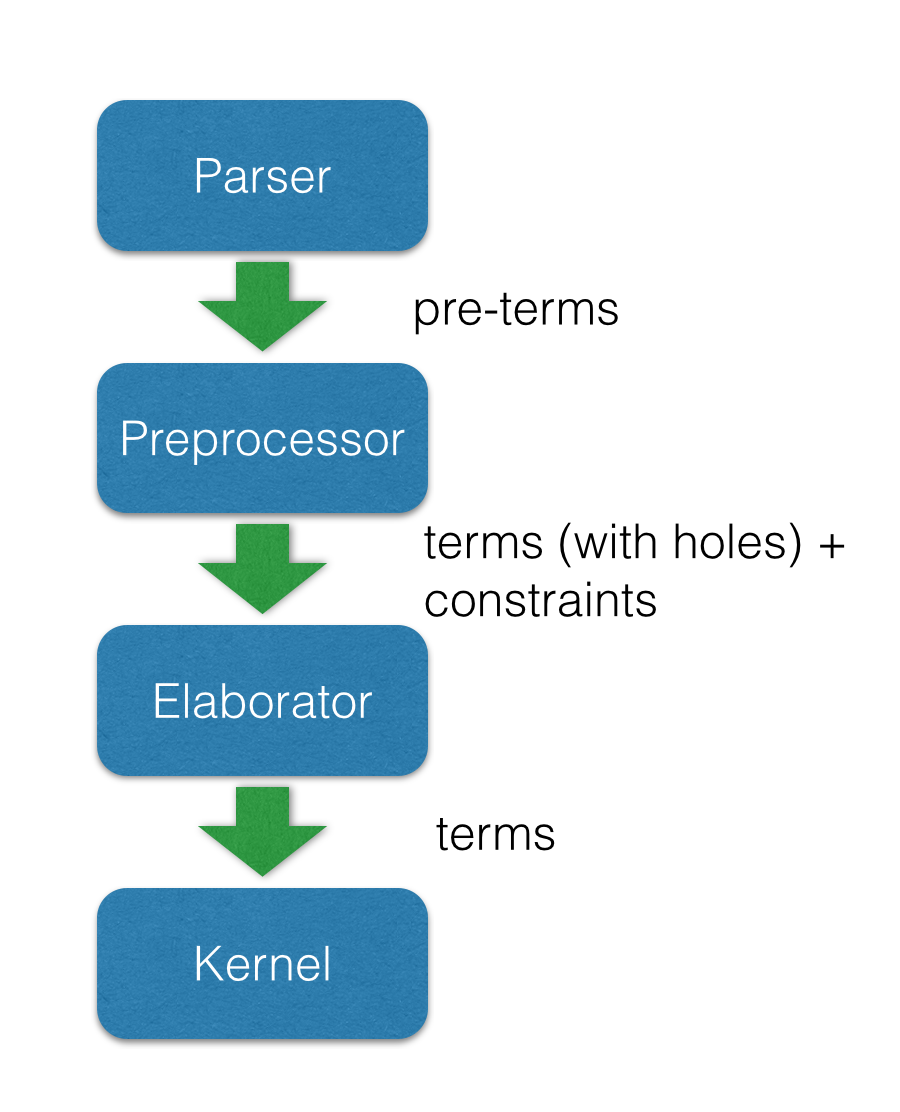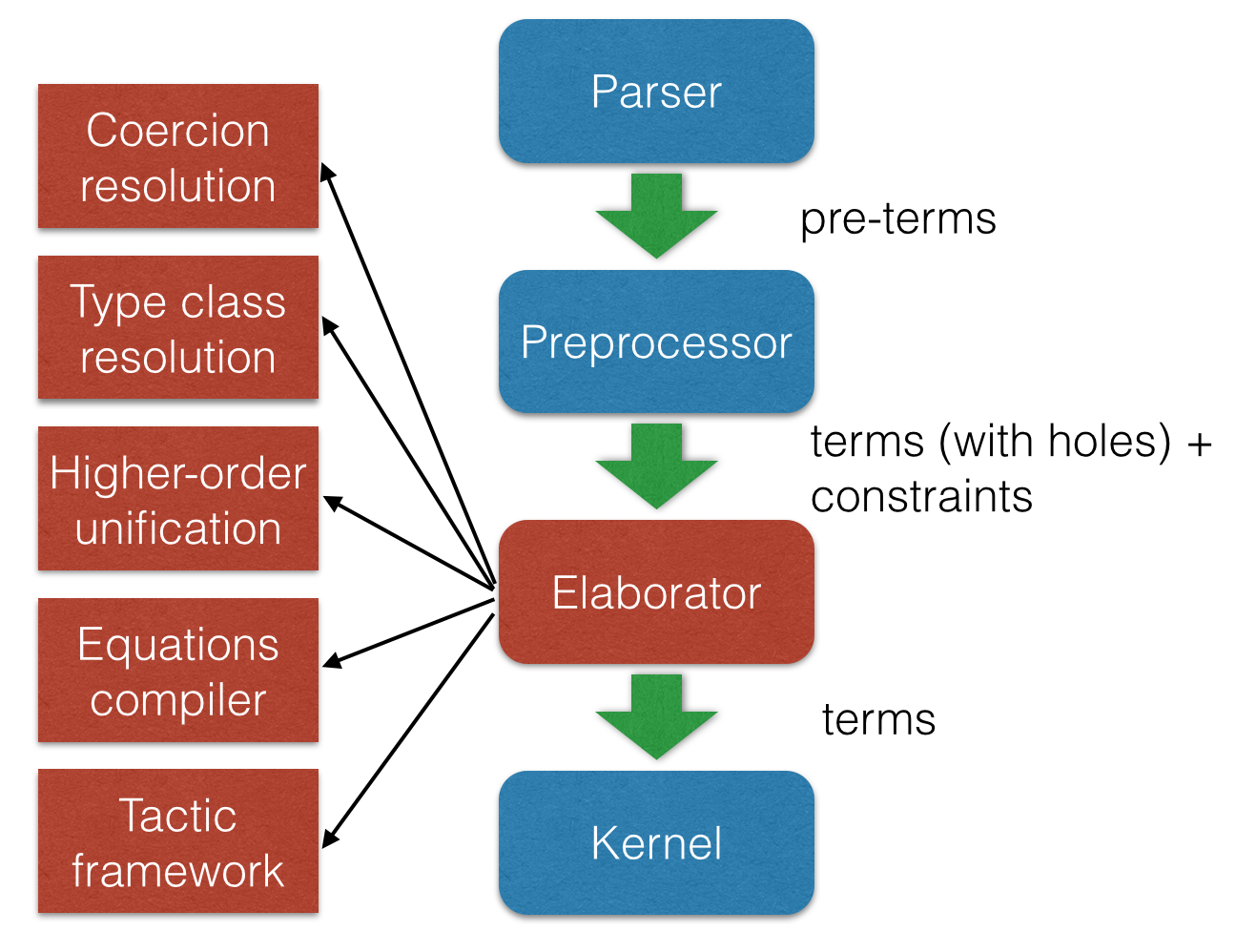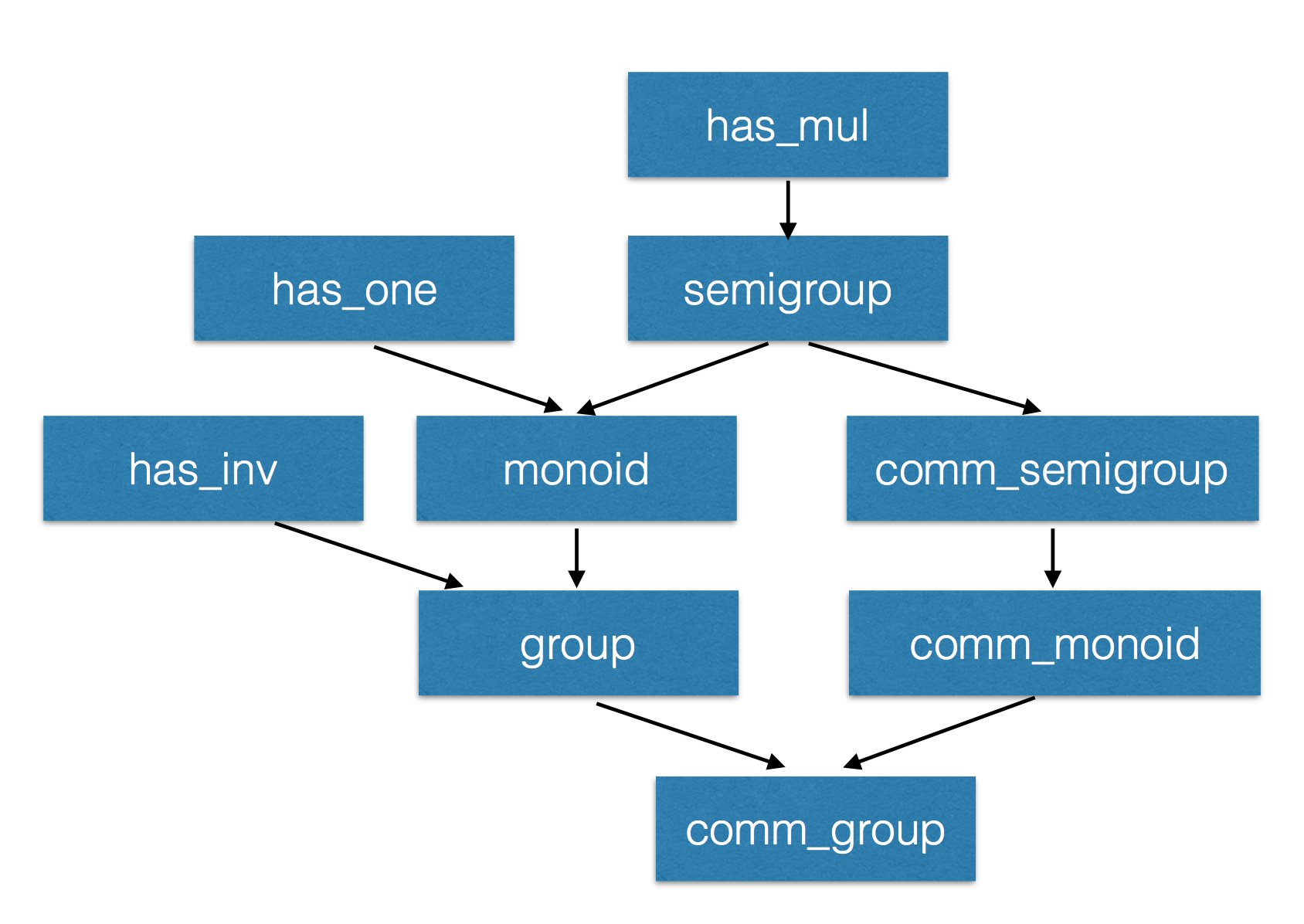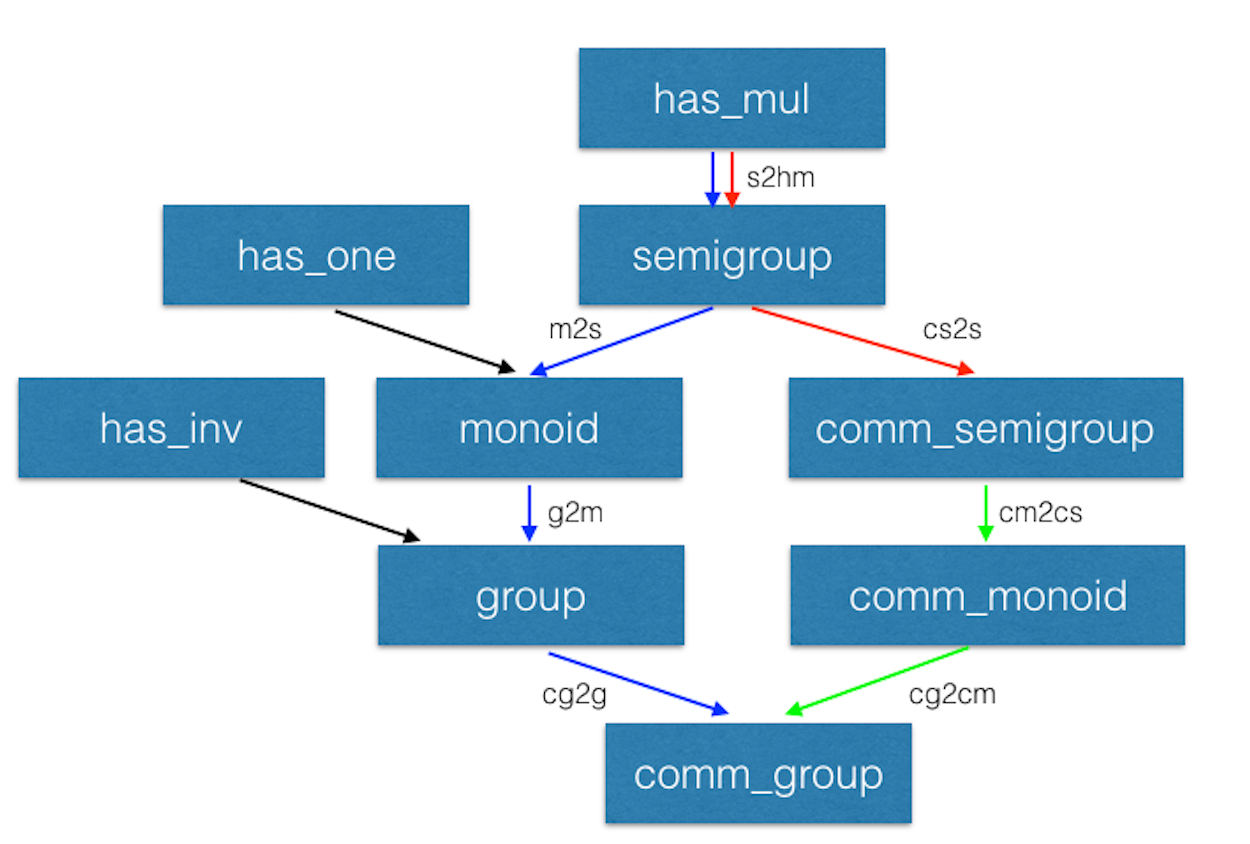# The Lean Theorem Prover

## The Lean Theorem Prover Team

• Gabriel Ebner (Vienna University of Technology),
• Sebastian Ullrich (Karlsruhe Institute of Technology),
• Floris van Doorn (CMU),
• Rob Lewis (CMU),
• Jared Roesch (UW),
• Daniel Selsam (Stanford)

## Many thanks to

• Soonho Kong
• Jakob von Raumer
• Cody Roux
• Georges Gonthier
• Grant Passmore
• Nikhil Swamy
• Assia Mahboubi
• Bas Spitters
• Steve Awodey
• Ulrik Buchholtz
• Tom Ball
• Parikshit Khanna
• Haitao Zhang

## Introduction: Lean

• New open source theorem prover
• Platform for
• Software verification & development
• Formalized mathematics
• Education (mathematics, logic, computer science)
• Synthesis (proofs & programs)
• de Bruijn's Principle: small trusted kernel
• Expressive logic
• Partial constructions: automation fills the "holes"
• Meta-programming

## Main Goal

Lean aims to bring two worlds together

• An interactive theorem prover with powerful automation
• An automated reasoning tool that
• produces (detailed) proofs,
• has a rich language,
• can be used interactively, and
• is built on a verified mathematical library

## Secondary Goals

• Robust proofs
• Minimalist and high-performace kernel
• Education
• Interactive courses
• Proving should be as easy as programming
• Have Fun

## Software verification and Formalized Mathematics

• Some projects at Microsoft Research
• Disclaimer: this projects were developed before Lean existed
• They used Boogie/Z3 and Coq.

## Software verification and Formalized Mathematics

• Similar problems
• Proof stability
• Libraries are big
• Scalability issues
• Finding existing functions/theorems
• Common problems in software engineering:
• Every attempt to create a single unified language failed (ADA?)
• We keep reimplementing the same libraries over and over again
• Mixing libraries from different languages is usually a mess
• Bit rotting
• These problems also affect formalized mathematics

## What is new?

• Meta-programming
• Extend Lean using Lean
• Proof/Program synthesizes
• Poweful elaboration engine that can handle
• Higher-order unification
• Type classes
• Coercions

"By relieving the brain of all unnecessary work, a good notation sets it free to concentrate on more advanced problems, and in effect increases the mental power of the race." – A. N. Whitehead

## What is new?

• Meta-programming
• Poweful elaboration engine that can handle
• Small trusted kernel
• It does not contain
• Termination checker
• Fixpoint operators
• Pattern matching
• Module management

## What is new?

• Meta-programming
• Poweful elaboration engine that can handle
• Small trusted kernel
• Multi-core support
• Process theorems in parallel
• Execute/try tactics (automation) in parallel

## What is new?

• Meta-programming
• Poweful elaboration engine that can handle
• Small trusted kernel
• Multi-core support
• Fast incremental compilation

## What is new?

• Meta-programming
• Poweful elaboration engine that can handle
• Small trusted kernel
• Multi-core support
• Fast incremental compilation
• Support for mixed declarative and tactic proof style

## Dependent Type Theory

• Before we started Lean, we have studied different theorem provers: ACL2, Agda, Automath, Coq, HOL (family), Isabelle, Mizar, PVS
• Dependent type theory is really beautiful
• Bultin computational interpretation
• Same data-structure for representing proofs and terms
• Reduce code duplication, example:
• We implemented a compiler for Haskell-like recursive equations, we can use it to construct proofs by induction
• Mathematical structures (such as Groups and Rings) are first-class citizens
• Some references

## Architecture## Architecture## The Logical Framework

• Dependent lambda calculus
Type -- Sort nat -- Constant λ x : nat, x -- Lambda abstraction vector bool 3 -- Application Π (n : nat), vector nat n -- Function Space nat → bool -- Function Space (no dependency)

## The Logical Framework

def f (A B : Type) : A → B → A := λ (a : A) (b : B), a def f (A B : Type) (a : A) (b : B) : A := a

## The Logical Framework

• Hierarchy of universes
check Prop -- Prop : Type 1 check Type 0 -- Prop : Type 1 check Type -- Type 1 : Type 2 check Type 1 -- Type 1 : Type 2 check Type 2 -- Type 2 : Type 3
• Universe polymorphic definitions
def {u} f (A B : Type u) : A → B → A := λ (a : A) (b : B), a

## The Logical Framework

• We "believe" in recursion
• Inductive families

inductive vector (A : Type) : nat → Type | nil : vector zero | cons : Π {n : nat}, A → vector n → vector (succ n)

## Inductive families

• Given

inductive nat : Type | zero : nat | succ : nat → nat
• Produces

-- 1) A new type check nat -- 2) Introduction rules (aka Constructors) check nat.zero check nat.succ -- 3) Eliminator (aka Recursor) check @nat.rec -- 4) Computational rule variable C : nat → Type variable Hz : C nat.zero variable Hs : Π (a : nat), C a → C (nat.succ a) eval nat.rec Hz Hs nat.zero -- Hz variable a : nat eval nat.rec Hz Hs (nat.succ a) -- Hs a (nat.rec Hz Hs a)

## Inductive families

def pred (a : nat) : nat := nat.rec nat.zero (λ (a₁ : nat) (r : nat), a₁) a eval pred (nat.succ (nat.succ nat.zero)) -- => nat.succ nat.zero

## Agnostic Mathematics

• Support constructive and classical mathematics
• Computation is important to mathematics
• Core parts of the standard library are constructive
• Separation of concerns:
• Methods to write computer programs
• Freedom to use a nonconstructive theories and methods to reason about them

## Noncomputable Keyword

• Our conjecture: most users don't care about constructivism, what they really care about is whether code can be generated or not for a definition.
private noncomputable def pb {s : seq} (Hs : regular s) (Hpos : pos s) := some (abs_pos_of_nonzero Hs (sep_zero_of_pos Hs Hpos)) private noncomputable def ps {s : seq} (Hs : regular s) (Hsep : sep s zero) := some (abs_pos_of_nonzero Hs Hsep) noncomputable def s_inv {s : seq} (Hs : regular s) (n : ℕ+) : ℚ := if H : sep s zero then (if n < (ps Hs H) then 1 / (s ((ps Hs H) * (ps Hs H) * (ps Hs H))) else 1 / (s ((ps Hs H) * (ps Hs H) * n))) else 0

## Freedom to trust

• Option: type check imported modules.
• Macros: semantic attachments for speeding up type checking and evaluation.
• Macros can be eliminated (expanded into pure Lean code).
• Each macro provides a function for computing the type and evaluating an instance.
• Each macro can be assigned a trust level.
• Many applications: interface with the GNU multiprecision arithmetic (GMP) library.

## Freedom to trust

• Relaxed mode
• Trust the imported modules have not been tampered
• Trust all macros
• Paranoid mode
• Retype check all imported modules (someone may have changed the binaries)
• Expand all macros (the developers may have made mistakes, GMP may be buggy)
• Stronger guarantee Retype check everything using Lean reference type checker

## Recursive equations

• Recursors are inconvenient to use.
• Compiler from recursive equations to recursors.
• Two compilation strategies: structural and well-founded recursion
def fib : nat → nat | 0 := 1 | 1 := 1 | (a+2) := fib (a+1) + fib a example (a : nat) : fib (a+2) = fib (a+1) + fib a := rfl
• Proofs by induction

## Recursive equations

• Dependent pattern matching
def map {A B C : Type} (f : A → B → C) : Π {n : nat}, vector A n → vector B n → vector C n | nil nil := nil | (a::va) (b::vb) := f a b :: map va vb def zip {A B : Type} : Π {n}, vector A n → vector B n → vector (A × B) n | nil nil := nil | (a::va) (b::vb) := (a, b) :: zip va vb

variables {ob₁ ob₂ : Type u} {C : category ob₁} {D : category ob₂} {F G H : C ⇒ D} def nt_compose (η : G ⟹ H) (θ : F ⟹ G) : F ⟹ H := natural_transformation.mk (take a, η a ∘ θ a) (take a b f, calc H f ∘ (η a ∘ θ a) = (H f ∘ η a) ∘ θ a : assoc ... = (η b ∘ G f) ∘ θ a : naturality ... = η b ∘ (G f ∘ θ a) : assoc ... = η b ∘ (θ b ∘ F f) : naturality ... = (η b ∘ θ b) ∘ F f : assoc

## Type classes

class has_sizeof (A : Type u) := (sizeof : A → nat) variables {A : Type u} {B : Type v} def sizeof [has_sizeof A] : A → nat instance : has_sizeof nat := ⟨λ a : nat, a⟩ -- ⟨...⟩ is the anonymous constructor instance [has_sizeof A] [has_sizeof B] : has_sizeof (prod A B) := ⟨λ p, match p with | (a, b) := sizeof a + sizeof b + 1 end⟩ instance [has_sizeof A] [has_sizeof B] : has_sizeof (sum A B) := ⟨λ s, match s with | inl a := sizeof a + 1 | inr b := sizeof b + 1 end⟩ vm_eval sizeof (10, 20) -- 31

## Type classes

class inhabited (A : Type u) := (default : A) class inductive decidable (p : Prop) | is_false : ¬p → decidable | is_true : p → decidable class has_one (A : Type u) := (one : A) class has_add (A : Type u) := (add : A → A → A) class has_mul (A : Type u) := (mul : A → A → A) class semigroup (A : Type) extends has_mul A := (mul_assoc : ∀ a b c : A, a * b * c = a * (b * c)) class monoid (A : Type) extends semigroup A, has_one A := (one_mul : ∀ a : A, 1 * a = a) (mul_one : ∀ a : A, a * 1 = a) class functor (F : Type u → Type v) := (map : Π {A B : Type u}, (A → B) → F A → F B) class monad (M : Type u → Type v) extends functor M := (ret : Π {A : Type u}, A → M A) (bind : Π {A B : Type u}, M A → (A → M B) → M B)

## Coercions as type classes

class has_coe (A : Type u) (B : Type v) := (coe : A → B) instance coe_bool_to_Prop : has_coe bool Prop := ⟨λ b, b = tt⟩ structure subtype {A : Type u} (p : A → Prop) := (elt_of : A) (has_property : p elt_of) instance coe_subtype {A : Type u} {p : A → Prop} : has_coe (subtype p) A := ⟨λ s : subtype, elt_of s⟩ def below (n : nat) : nat → Prop := λ i, i < n def f {n : nat} (v : subtype (below n)) : nat := v + 1 --^ Coercion used here

## Meta-programming

• Extending Lean in Lean
• Lean has an efficient bytecode interpreter

inductive expr | var : unsigned → expr | sort : level → expr | const : name → list level → expr | app : expr → expr → expr ... meta constant tactic_state : Type inductive tactic_result (A : Type) | success : A → tactic_state → tactic_result | exception : (unit → format) → tactic_state → tactic_result meta def tactic (A : Type) := tactic_state → tactic_result A meta instance : monad tactic := ... meta constant infer_type : expr → tactic expr meta constant subst : expr → tactic unit meta constant exact : expr → tactic unit meta def apply : expr → tactic unit := ...

## Meta-programming

meta definition expr_of_nat : nat → tactic expr | 0 := to_expr (0) | 1 := to_expr (1) | n := do r ← expr_of_nat (n / 2), if n % 2 = 0 then to_expr (bit0 %%r) else to_expr (bit1 %%r) run_command do r ← expr_of_nat 10, trace r -- bit0 (bit1 (bit0 one))

## Meta-programming (decidable equality)

• Building an equality predicate for each new type is very tedious.

def decidable_eq (A : Type u) := ∀ (a b : A), decidable (a = b) instance nat_dec_eq : decidable_eq ℕ | zero zero := is_true rfl | (succ x) zero := is_false (λ h, nat.no_confusion h) | zero (succ y) := is_false (λ h, nat.no_confusion h) | (succ x) (succ y) := match nat_dec_eq x y with | is_true xeqy := is_true (xeqy ▸ eq.refl (succ x)) | is_false xney := is_false (λ h, nat.no_confusion h (λ xeqy, absurd xeqy xney)) end
• We implemented a tactic in Lean (< 100 lines) that creates these instances automatically.

variables {A : Type u} {B : Type v} instance {p : A → Prop} [decidable_eq A] : decidable_eq (subtype p) := by mk_dec_eq_instance instance [decidable_eq A] : decidable_eq (list A) := by mk_dec_eq_instance instance [decidable_eq A] [decidable_eq B] : decidable_eq (sum A B) := by mk_dec_eq_instance

## Meta-programming (examples)

• Resolution prover (Gabriel Ebner)

structure resolution_prover_state := (active : rb_map name active_cls) (passive : rb_map name cls) (newly_derived : list cls) (prec : list expr) (age : nat) meta def resolution_prover := stateT resolution_prover_state tactic
• Isabelle's auto tactic (Jeremy Avigad)

## Simplifier

meta def simp : tactic unit := ... example (a b : nat): a + 0 + b = b + a := by simp @[simp] lemma (x : nat) : f x x = 0 := ... example (a b : nat) : f a a + b = b := by simp

## Structures

• Simplifier applies generic lemmas.
universe variable u class ring (A : Type u) extends add_comm_group A, monoid A, distrib A variable {A : Type u} theorem ring_mul_zero [ring A] (a : A) : a * 0 = 0 := have a * 0 + 0 = a * 0 + a * 0, from calc a * 0 + 0 = a * 0 : by simp ... = a * (0 + 0) : by simp ... = a * 0 + a * 0 : by simp show a * 0 = 0, by simp instance : ordered_ring ℝ := ... example (x : real) : x * 0 = 0 := ring_mul_zero x

universe variable u class ring (A : Type u) extends add_comm_group A, monoid A, distrib A variable {A : Type u} theorem ring_mul_zero [ring A] (a : A) : a * 0 = 0 := ... theorem ring_zero_mul [ring A] (a : A) : 0 * a = 0 := ... instance ring_to_semiring [s : ring A] : semiring A := { s with mul_zero := ring_mul_zero, zero_mul := ring_zero_mul }

## Diamondsvariables {A : Type} theorem right_comm [s : comm_semigroup A] (a b c : A) : (a * b) * c = (a * c) * b := -- In the theorem above, (a * b) is notation for -- @mul A (semigroup_to_has_mul A (comm_semigroup_to_semigroup A s)) a b

## Diamonds (cont.)

• Now, given a [g : comm_group A], suppose we want to apply the theorem right_comm to the following term as a rewriting rule.
variable [g : comm_group A] variables x y z : A (x * y) * (x * y) -- That is, we want to match the pattern (?a * ?b) * ?c -- with (x * y) * (x * y)
• What about the argument [s : comm_semigroup A]?

## Diamonds (cont.)

• When we expand the notation, we can see the "hidden" complexity. For simplicity, let's assume we are trying to match ?a * ?b with x * y
@mul ?A (s2hm ?A (cs2s ?A ?s)) ?a ?b -- with @mul A (s2hm A (m2s A (g2s A (cg2g A g)))) x y -- Lean finds the solution ?A := A; ?s := (cm2cs A (cg2cm A g)); ?a := x; ?b := y## Work in progress

• C++ code generation (Jared Roesch - UW)
• Many of the compilation steps are shared with the bytecode interpreter.
• Application 1: efficient tactics
• Write tactic/automation in Lean
• Generate C++ code
• Build shared library and dynamically link with Lean executable
• Application 2: low-level hacking
• Foreign function interface

## Work in progress

• AC rewriting
• SMT-like automation
• Main challenge: dependent types
• Daniel Selsam and I have developed a congruence closure procedure and E-matching for dependent type theory (IJCAR 2016).
• Extending the parser using Lean